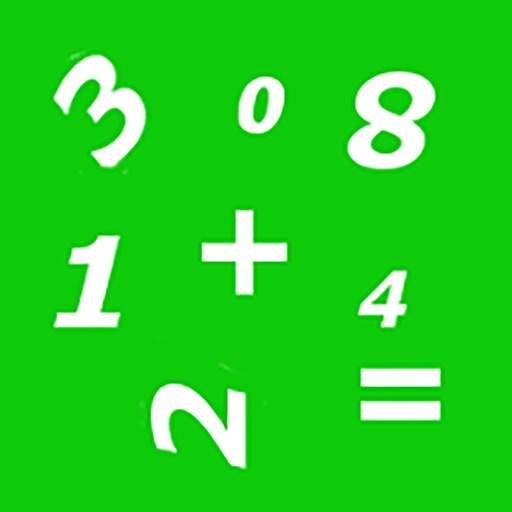## The puzzle game of Mathoku is similar to Sudoku but with a mathematical twist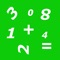# Mathoku

by Kairos Development Co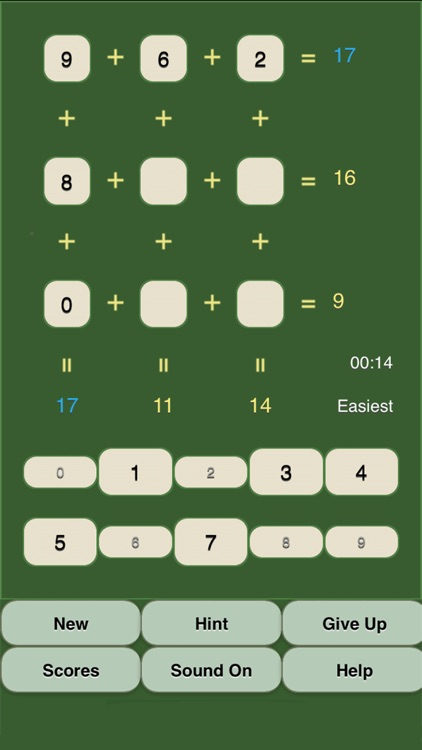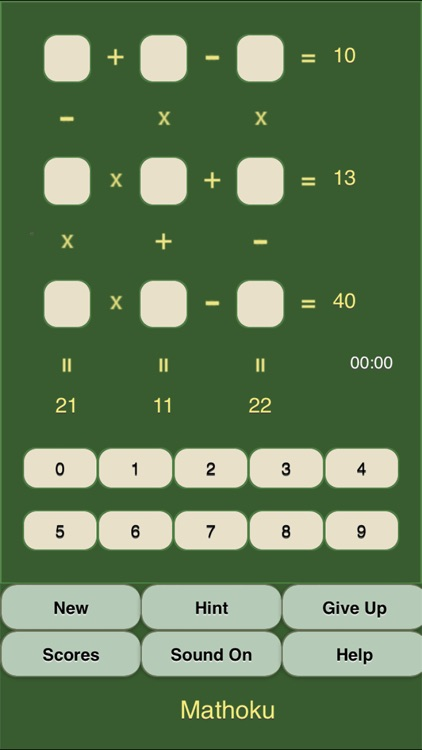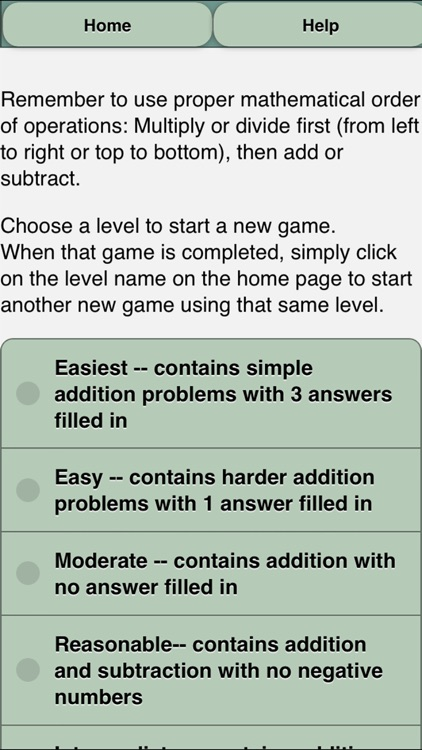The puzzle game of Mathoku is similar to Sudoku but with a mathematical twist. The goal is to place numbers from zero to nine in the spaces to produce the correct total for each row and column, utilizing the mathematical operations shown.### App Details

Version
3.1
Rating
(4)
Size
29Mb
Genre
Strategy Puzzle Education
Last updated
May 29, 2019
Release date
April 21, 2011

### App Screenshots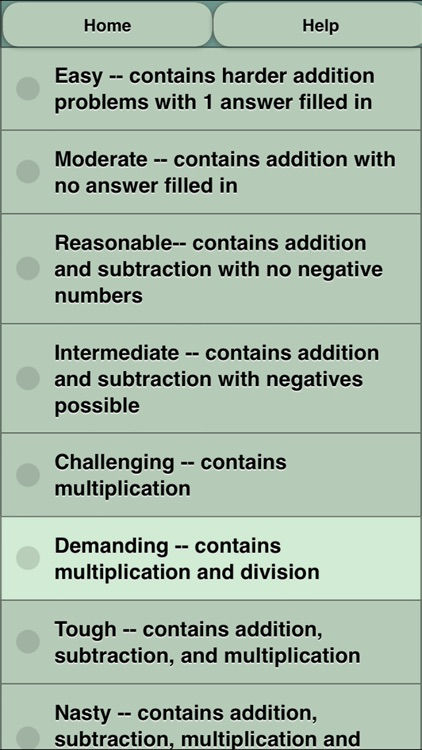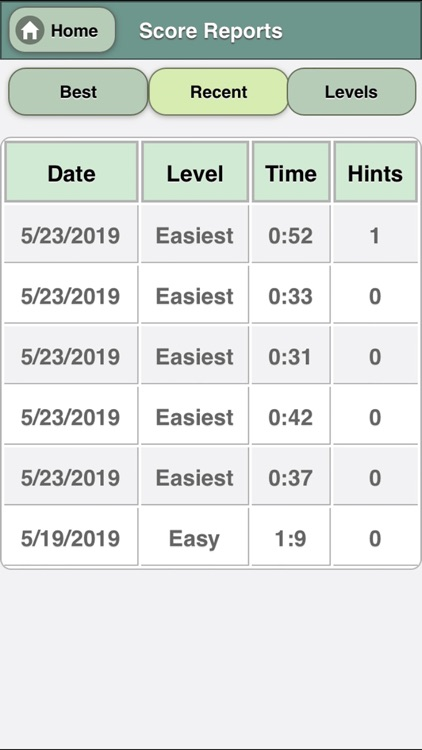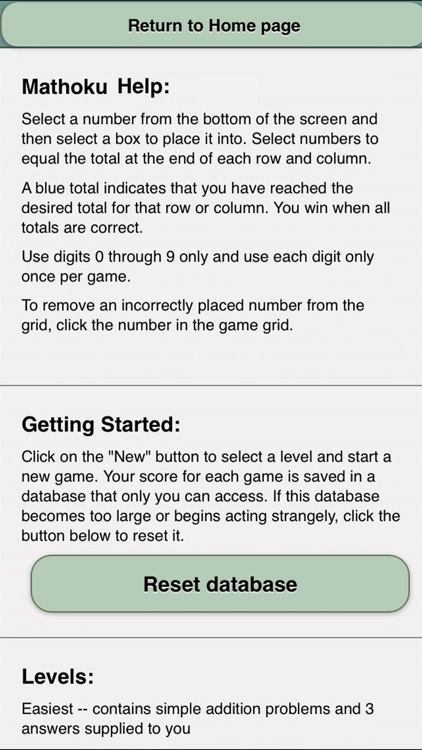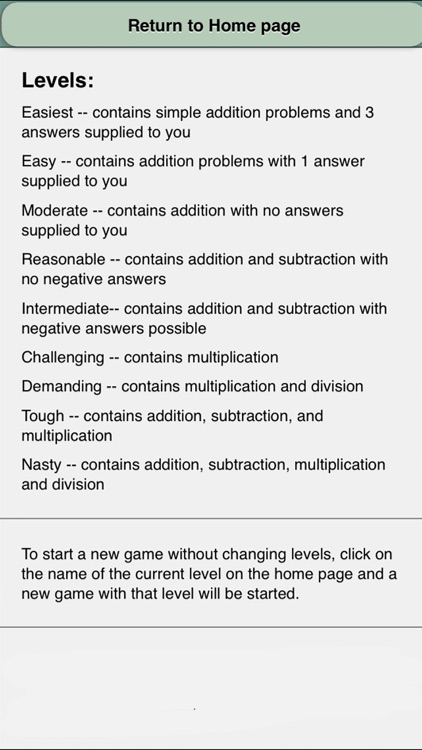### App Store Description

The puzzle game of Mathoku is similar to Sudoku but with a mathematical twist. The goal is to place numbers from zero to nine in the spaces to produce the correct total for each row and column, utilizing the mathematical operations shown.

Mathoku is easy to learn and very addictive. Since the app utilizes random numbers to generate each puzzle, the number of combinations is almost endless (over 2 million games per level).

New in this version is the ability to save scores and view reports of those scores.

Created by a certified Math teacher, Mathoku can help sharpen a child’s arithmetic and mathematical skills as well as stimulate the logical mathematical minds of young adults. Fans of Sudoku, Twenty-four and Kenken will love it.

There are five difficulty levels and the ability to ask for a hint when needed. The mathematical rules of order of operations are reinforced with this app.

Challenge yourself today!